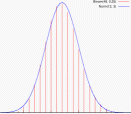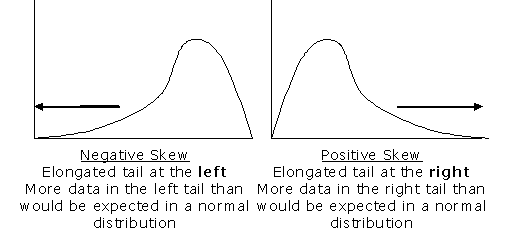Email us to get an instant 20% discount on highly effective K-12 Math & English kwizNET Programs!

#### Online Quiz (WorksheetABCD)

Questions Per Quiz = 2 4 6 8 10

### High School Mathematics - 211.9 Normal and Skewed Distributions

 Normal and skewed distributions: The probability distributions we studied so far are discrete probability distributions because they have only a finite number of possible values. A discrete probability distribution can be represented by a histogram. For a continuous probability distribution, the outcome can be any value in an interval of real numbers. Continuous probability distributions are represented by curves instead of histograms. Such curves are often called a bell curve. Many distributions with symmetric curves or histograms are normal distributions. In normal distributions scores are more concentrated in the middle than in the tails.A curve or histogram that is not symmetric represents a skewed distribution. Positive skew: The right tail is longer and the mass of the distribution is concentrated on the left of the figure. The distribution is said to be right-skewed. Negative skew: The left tail is longer and the mass of the distribution is concentrated on the right of the figure. The distribution is said to be left-skewed.Q 1: Distributions that are symmetric are called _________ distributions.Answer: Q 2: Determine whether the data 14, 15, 11, 13, 13, 14, 15, 14, 12, 13, 14, 15 appear to be positively skewed, negatively skewed, or normal distributed.Answer: Question 3: This question is available to subscribers only! Question 4: This question is available to subscribers only!